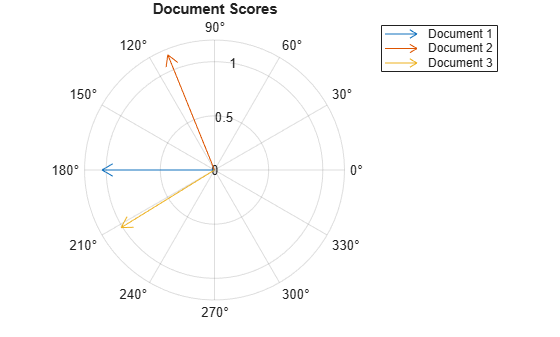# lsaModel

Latent semantic analysis (LSA) model

## Description

A latent semantic analysis (LSA) model discovers relationships between documents and the words that they contain. An LSA model is a dimensionality reduction tool useful for running low-dimensional statistical models on high-dimensional word counts. If the model was fit using a bag-of-n-grams model, then the software treats the n-grams as individual words.

## Creation

Create an LSA model using the `fitlsa` function.

## Properties

expand all

Number of components, specified as a nonnegative integer. The number of components is the dimensionality of the result vectors. Changing the value of `NumComponents` changes the length of the resulting vectors, without influencing the initial values. You can only set `NumComponents` to be less than or equal to the number of components used to fit the LSA model.

Example: 100

Exponent scaling feature component strengths for the `DocumentScores` and `WordScores` properties, and the `transform` function, specified as a nonnegative scalar. The LSA model scales the properties by their singular values (feature strengths), with an exponent of `FeatureStrengthExponent/2`.

Example: 2.5

Component weights, specified as a numeric vector. The component weights of an LSA model are the singular values, squared. `ComponentWeights` is a 1-by-`NumComponents` vector where the jth entry corresponds to the weight of component j. The components are ordered by decreasing weights. You can use the weights to estimate the importance of components.

Score vectors per input document, specified as a matrix. The document scores of an LSA model are the score vectors in lower dimensional space of each document used to fit the LSA model. `DocumentScores` is a D-by-`NumComponents` matrix where D is the number of documents used to fit the LSA model. The (i,j)th entry of `DocumentScores` corresponds to the score of component j in document i.

Word scores per component, specified as a matrix. The word scores of an LSA model are the scores of each word in each component of the LSA model. `WordScores` is a V-by-`NumComponents` matrix where V is the number of words in `Vocabulary`. The (v,j)th entry of `WordScores` corresponds to the score of word v in component j.

Unique words in the model, specified as a string vector.

Data Types: `string`

## Object Functions

 `transform` Transform documents into lower-dimensional space

## Examples

collapse all

Fit a Latent Semantic Analysis model to a collection of documents.

Load the example data. The file `sonnetsPreprocessed.txt` contains preprocessed versions of Shakespeare's sonnets. The file contains one sonnet per line, with words separated by a space. Extract the text from `sonnetsPreprocessed.txt`, split the text into documents at newline characters, and then tokenize the documents.

```filename = "sonnetsPreprocessed.txt"; str = extractFileText(filename); textData = split(str,newline); documents = tokenizedDocument(textData);```

Create a bag-of-words model using `bagOfWords`.

`bag = bagOfWords(documents) `
```bag = bagOfWords with properties: Counts: [154x3092 double] Vocabulary: [1x3092 string] NumWords: 3092 NumDocuments: 154 ```

Fit an LSA model with 20 components.

```numComponents = 20; mdl = fitlsa(bag,numComponents)```
```mdl = lsaModel with properties: NumComponents: 20 ComponentWeights: [1x20 double] DocumentScores: [154x20 double] WordScores: [3092x20 double] Vocabulary: [1x3092 string] FeatureStrengthExponent: 2 ```

Transform new documents into lower dimensional space using the LSA model.

```newDocuments = tokenizedDocument([ "what's in a name? a rose by any other name would smell as sweet." "if music be the food of love, play on."]); dscores = transform(mdl,newDocuments)```
```dscores = 2×20 0.1338 0.1623 0.1680 -0.0541 -0.2464 -0.0134 -0.2604 -0.0205 -0.1127 0.0627 0.3311 -0.2327 0.1689 -0.2695 0.0228 0.1241 0.1198 0.2535 -0.0607 0.0305 0.2547 0.5576 -0.0095 0.5660 -0.0643 -0.1236 0.0082 0.0522 0.0690 -0.0330 0.0385 0.0803 -0.0373 0.0384 -0.0005 0.1943 0.0207 0.0278 0.0001 -0.0469 ```

Create a bag-of-words model from some text data.

```str = [ "I enjoy ham, eggs and bacon for breakfast." "I sometimes skip breakfast." "I eat eggs and ham for dinner." ]; documents = tokenizedDocument(str); bag = bagOfWords(documents);```

Fit an LSA model with two components. Set the feature strength exponent to 0.5.

```numComponents = 2; exponent = 0.5; mdl = fitlsa(bag,numComponents, ... 'FeatureStrengthExponent',exponent)```
```mdl = lsaModel with properties: NumComponents: 2 ComponentWeights: [16.2268 4.0000] DocumentScores: [3x2 double] WordScores: [14x2 double] Vocabulary: [1x14 string] FeatureStrengthExponent: 0.5000 ```

Calculate the cosine distance between the documents score vectors using `pdist`. View the distances in a matrix `D` using `squareform`. `D(i,j)` denotes the distance between document `i` and `j`.

```dscores = mdl.DocumentScores; distances = pdist(dscores,'cosine'); D = squareform(distances)```
```D = 3×3 0 0.6244 0.1489 0.6244 0 1.1670 0.1489 1.1670 0 ```

Visualize the similarity between documents by plotting the document score vectors in a compass plot.

```figure compass(dscores(1,1),dscores(1,2),'red') hold on compass(dscores(2,1),dscores(2,2),'green') compass(dscores(3,1),dscores(3,2),'blue') hold off title("Document Scores") legend(["Document 1" "Document 2" "Document 3"],'Location','bestoutside')```Introduced in R2017b